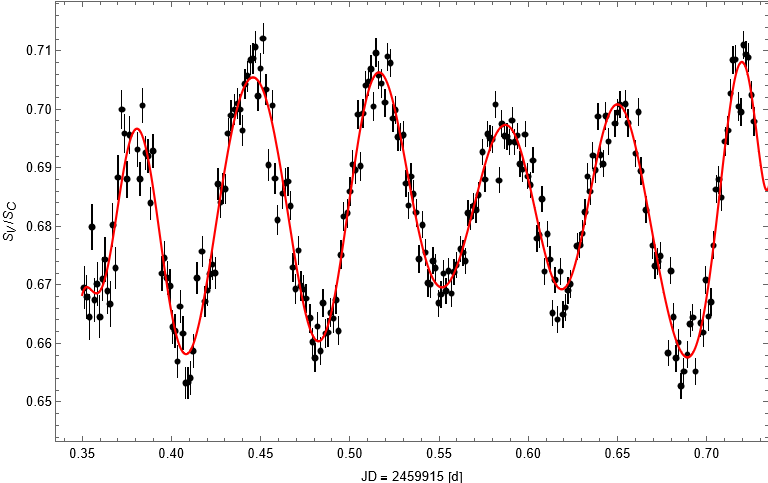# V 0750 Aur

Frederik Krenzer & Jonathan Laux

In our observation and investigation of the variable star V 0750 Aur in the constellation Auriga (see figure 1) on december 1st 2022, we could detect not one, but at least two different pulsation periods which we calculated to be 0.0678 d (97.6 minutes) and 0.274 d (6.58 hours).Here, one can see the measured light curve, it can be seen that these changes in brightness cannot be described by just one frequency, but rather two ore more frequencies corresponding to different pulsation cycles. Multiple types of oscillation seem to coexist in this case. We tried to use a Fourier-model to determine the frequencies, the resulting funtction is also shown in figure 2. While the graph seems to fit the data quite well, the calculated frequencies did not represent the visible oscillations. The model gives a much lower primary frequency while the visible oscillations are represented by higher orders in the fourier expansion. We extracted the two most dominant frequencies directly from the graph instead. For a variable star as complex as this one, other mathematical models might give better results.

When we compared our results with the values given in the Variable Star Index VSX, we found just one of the frequencies we calculated, with a deviation of about 8%. Additionally, two more frequencies were observed in the past. It seems as if various complex mechanisms are responsible for the changes in brightness of V 0750 Aur, and one observation is not enough to fully describe its behavior.